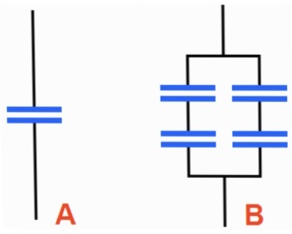# Problem: The two configurations shown below are constructed using identical capacitors. 1) Which of these configurations has the lowest overall capacitance? a) A b) B c) Both configurations have the same capacitance2) Briefly explain your reasoning

###### FREE Expert Solution

Equivalent capacitance in series:

$\overline{)\frac{\mathbf{1}}{{\mathbf{C}}_{\mathbf{e}\mathbf{q}}}{\mathbf{=}}\frac{\mathbf{1}}{{\mathbf{C}}_{\mathbf{1}}}{\mathbf{+}}\frac{\mathbf{1}}{{\mathbf{C}}_{\mathbf{2}}}}$

Equivalent capacitance in parallel:

$\overline{){{\mathbf{C}}}_{\mathbf{e}\mathbf{q}}{\mathbf{=}}{{\mathbf{C}}}_{{\mathbf{1}}}{\mathbf{+}}{{\mathbf{C}}}_{{\mathbf{2}}}}$

(1)

85% (296 ratings)###### Problem Details

The two configurations shown below are constructed using identical capacitors.1) Which of these configurations has the lowest overall capacitance?

a) A

b) B

c) Both configurations have the same capacitance